﻿ Mechanical Model of Hydrogen Bonds in Protein Molecules

### Mechanical Model of Hydrogen Bonds in Protein Molecules

Zahra ShahbaziOPEN ACCESSPEER-REVIEWED

## Mechanical Model of Hydrogen Bonds in Protein Molecules

Zahra ShahbaziDepartment of Mechanical Engineering, Manhattan College, NY

### Abstract

The unique properties of protein molecules have motivated researchers exploit them in the design and fabrication of bio-mimetic nano devices to perform a special task. Function of protein molecules is in turn dependent on their 3D structure and their ability to modify their shape for a specific task. To study and manipulate protein molecules we need to have knowledge of mechanical properties of these molecules. In this paper a multiscale model to predict stiffness of helical protein molecules has been developed. Hydrogen bonds as major contributing factor to proteins flexibility, are modeled as elastic springs based on their empirical potential energy. Such mechanical representation of hydrogen bonds enables us to obtain the stiffness ellipsoid of hydrogen bonds which leads to an understanding of the directional stiffness of protein molecules. The model has also been applied to three different protein molecules whose stiffness were reported in the literature. The comparison shows an agreement between the stiffness computed by the proposed model and that obtained through experiments and/or Molecular Dynamics (MD) simulations.

### At a glance: Figures

12
Prev Next

• Shahbazi, Zahra. "Mechanical Model of Hydrogen Bonds in Protein Molecules." American Journal of Mechanical Engineering 3.2 (2015): 47-54.
• Shahbazi, Z. (2015). Mechanical Model of Hydrogen Bonds in Protein Molecules. American Journal of Mechanical Engineering, 3(2), 47-54.
• Shahbazi, Zahra. "Mechanical Model of Hydrogen Bonds in Protein Molecules." American Journal of Mechanical Engineering 3, no. 2 (2015): 47-54.

 Import into BibTeX Import into EndNote Import into RefMan Import into RefWorks

### 1. Introduction

Unique physical properties of biomaterials such as self-assembly, self-healing, adaptability and changeability have encouraged researchers to devote special attention to designing and fabricating synthetic materials that have similar properties [1, 2, 3]. These unique properties are facilitated by building blocks of biomaterials, proteins. In order to understand the properties of proteins, one has to initially understand their structure. This structure can be considered on several levels, all of which influence the final property and behavior of the material.

Previous efforts of finding the stiffness of protein molecules can be classified into two major categories of experimental studies and computational analysis. Regarding experimental efforts, recently Atomic Force Microscopy (AFM) has been used for studying mechanical properties of protein molecules. This technique measures the force required to stretch individual molecules and calculates its stiffness [4, 5, 6, 7]. Laser tweezer is another experimental technique used to analyze material properties in molecular level . Researchers also conducted computational simulations to predict mechanical properties of protein molecules [9, 10, 11].

Experimental studies tend to increase in difficulty as the size of the molecule becomes smaller. Existing computational efforts such as molecular dynamics, on the other hand, are more computationally expensive as the molecules become larger . Such size dependency reveals the inherent difficulty of finding the mechanical properties of protein molecules.

Here we seek to establish the relation of the structure at its atomic length scale to the mechanical properties of protein molecules. To do this, we started with the interatomic bonds. In most protein molecules, hydrogen bonds are the key contributor to the molecules’ stiffness. Therefore, we have developed a computational approach that models each hydrogen bond as a novel three-spring system. Using an already existing empirical interatomic potential for the hydrogen bonds, the stiffness ellipsoid is developed which can provide us with the stiffness of each bond at any given direction. As the next step, we have investigated the fragility of extending the analysis to the entire protein molecule to find its stiffness. To verify our proposed method, three protein molecules whose stiffness values have already been reported in literature using alternative methods described earlier, are investigated and the results show a reasonable agreement suggesting the feasibility of the technique for larger protein molecules.

A more comprehensive detail of part of the work presented here can be found in . Additionally, a kinematic perspective of such modeling was also presented in 21st century kinematics workshop . The foundational work in the mechanical modeling of protein molecules can be found in references [15-21].

### 2. Hydrogen Bonds

Atoms in proteins are held together by various types of bonds with different strength including very strong covalent bonds, moderate hydrogen bonds and weak van der Waals bonds. Hydrogen bonds contribute significantly to the folding of the proteins into stable conformations. Under external stimulations hydrogen bonds can change their length and corresponding angles. This gives them more flexibility than covalent bonds . Therefore hydrogen bonds play an important role in determining mechanical properties of protein molecules and stiffness in particular.

2.1. Geometry

A hydrogen bond within a protein molecule is an interaction between an electronegative donor and a hydrogen that is covalently bonded to an acceptor atom from non-adjacent residues. The relative placement (position and orientation) of the acceptor atom with respect to the donor atom can be rigorously defined through a set of geometric parameters (angles and distances) . A unified set of geometric parameters is illustrated in Figure 1 where a donor (nitrogen), an acceptor (oxygen), acceptor antecedent, donor antecedent and hydrogen atoms are shown. The bond lengths are:

m: the covalent bond length between donor (nitrogen) and hydrogen

r: the hydrogen bond length between hydrogen and acceptor (oxygen)

d: the distance between donor and acceptor

and the angles are defined as:

α: between AA (acceptor antecedent), A (oxygen) and hydrogen (AA–A–H)

θ : between AA (acceptor antecedent), A (oxygen) and D (nitrogen) ( AA–A–D)

λ : between D (nitrogen), A(oxygen) and hydrogen ( D–A–H)

β: between D (nitrogen), hydrogen and A(oxygen) (D–H–A)

γ: between hydrogen, D (nitrogen) and A (oxygen) (H–D–A)

ε: between DD (donor antecedent), D (nitrogen) and A (oxygen) (DD–D–A).

Different geometric formation criteria have been developed to predict hydrogen bonds occurrence in the protein molecules . In this paper, in order to identify hydrogen bonds we used the suggested geometric criteria by  shown in Table 1.

#### Table 1. Geometric criteria 

2.2. Hydrogen Bond Energy

Hydrogen bond energy depends on the chemistry of the donor and acceptor atoms as well as their orientation. Based on the potential function used in “Dreiding” force field, () suggested a function (equation 1) to calculate hydrogen bond energy. In this energy function, constants are: v0 = 8 kCal/mol-1 and d0 = 2.8Å.(1)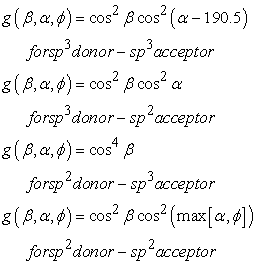Dahiyat energy function (equation 1) considers hydrogen bonds of protein molecules in any solvent. The energy values predicted by this function (due to both positional and angular coordinates) are commonly regarded in by other researchers in reasonable match with experimental data . Although, we are using  geometric criteria and  energy function for prediction of the stiffness of hydrogen bonds, the proposed method remains valid for any other method of predicting the bond geometry and its energy.

### 3. Proposed Hydrogen Bond Mechanical Model

Under the application of external forces on the molecule, or internal forces due to shape changes in the functional proteins, hydrogen bonds exhibit flexibility. Hydrogen bonds can tolerate ±0.17 Å change in bond length in average before breaking [25, 26, 27]. Therefore we modeled hydrogen bonds as flexible mechanical elements (linear springs). Our simulation is valid for small displacements ranging ± 0.12 Å. Considering the energy function of these bonds shown in equation 1 we modeled each individual hydrogen bond with specific geometry as a combination of three linear springs accurately estimating the bond energy change for small changes in the bond geometry. Consequently our model calculates the mechanical properties of the spring such as spring stiffness and spring free length (in three directions) for each individual hydrogen bond. This is the major achievement of this study. Previous mechanical model of hydrogen bonds considered the bond to be flexible solely in bond direction and assumed the same stiffness for all bonds with different geometry .

3.1. Equivalent Stiffens for Individual Hydrogen Bonds

To calculate stiffness of each bond the energy function of the bond (shown in equation 1) has been used. In this equation the energy is a function of three geometric parameters: donor-acceptor bond length (d), and the two angles (α&β) as shown in Figure 2-A. To simplify the model, angular coordinates (α&β) in energy function are replaced by the positional coordinates l and r, using geometric relations 2 and 3.(2)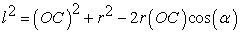(3)

Figure 2 B shows our proposed equivalent mechanical system to model hydrogen bonds. This model consists of three linear springs. The energy of this system should be the same as the energy of the bond itself computed by equation 1. Using Hook’s law the equivalent energy of the spring system is shown in equation 4.(4)

To fully define the model, we need to calculate the values of the six constants (kr, r0, kl, l0, kd, d0) in the right hand side of the equation 4. In order to obtain these constants, we have set up an optimization problem with the aim of minimizing the fitting error; that is the difference between the bond energy obtained by empirical studies (equation 1) and our proposed model (equation 4).

The optimization problem has the following constraints:

The energy computed at the configuration prior to the small displacements by both methods (equations 1 and 4) should be equal. This condition is implemented in equation 6.

The three spring constants (for the springs in Figure 2) are the slope of graph describing each spring force versus interatomic separation distance . These conditions are implemented in equations 7 to 9.

Stiffness and the free lengths of springs should be positive numbers (equations 10 to 15).

The optimization problem (minimizing the difference between the bond energy obtained by empirical studies and our proposed model after small displacement) is therefore summarized as follows:

Minimize M (the objective function)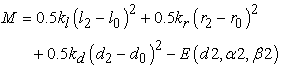(5)

Subject to the following constraints:(6)(7)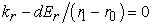(8)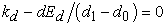(9)(10)(11)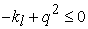(12)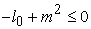(13)(14)(15)

This optimization problem is then solved using Lagrange Multipliers method . Figure 3 shows the energy of the hydrogen bond versus the bond length (r) obtained by empirical studies (dashed curve) and our optimized model (solid curve). As shown in Figure 3, the proposed function can properly estimate the empirical energy function for small variations of the bond length.

3.2. Equivalent Stiffness Ellipsoid

Equivalent stiffness ellipsoid Once we have the stiffness of three springs, modeling individual hydrogen bonds, we can calculate bond stiffness at any given direction. To do this, stiffness matrix and consequently stiffness ellipsoid (ellipsoid equation) for each bond is calculated using static equilibrium equations (16, 17 and 18) for the mechanical system shown in Figure 4. In this system link NH is assumed to be fixed and link OC is free to move (from O1C1 to O2C2). The free body diagram of the system is shown in Figure 4-B.(16)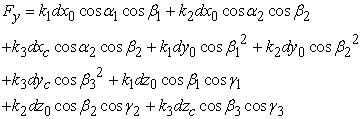(17)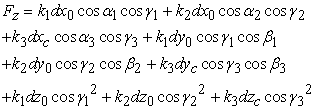(18)

Rearranging these equations, the stiffness matrix can be determined from equation 19.(19)(20)

The maximum and minimum stiffness of the system at point C, and their corresponding directions can be computed from stiffness matrix. The process is as follows. A unit displacement vector at point C is assumed. The corresponding force that is required to cause this displacement is calculated from equation 20. The process of finding the minimum and maximum possible force vectors is then setup as an optimization problem:It can be shown that these maximum and minimum forces are the eigenvectors of the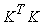matrix, with magnitudes of these forces being the square root of the corresponding eigenvalues. The three obtained eigenvalues are the corresponding diameters of the stiffness ellipsoid, and the eigen vectors are ellipsoid’s principal directions.

The stiffness of the bond at any other direction, Ut, can be computed by calculating the intersection of the stiffness ellipsoid and the line passing through the center of the ellipsoid in the Ut direction. Figure 5 shows a schematic presentation of stiffness ellipsoid and its orientation with respect to bond geometry.

### 4. Results and Model Validations

4.1. For Single Hydrogen Bond

The developed methodology in previous section has been applied on several sample hydrogen bonds. Table 1 provides an overview of the results, detailing the following parameters: (E) the bond energy (kCal/mol), (kl,kr&kd) stiffness (N/m) of each spring, (l0,r0&d0) free length of springs (Å), eigen values of stiffness matrix which are the maximum, mean and minimum stiffness of the bonds (N/m), (θ*) the angle between maximum stiffness direction and hydrogen bond direction (degrees), (k**) the hydrogen bond stiffness in bond (OH) direction (N/m). Table 3 lists stiffness for various bonds obtained from . Our reported stiffness for hydrogen bonds in Table 2, ranging from 1.19-5.74 N/m with an average of 3.43N/m and standard deviation of 1.3N/m, are in agreement with the empirical results reported by  (3-6N/m). It has been shown in Table 2, column 11 which hydrogen bonds’ maximum stiffness direction needs to be rotated 5 to 10 degrees to be aligned with bond direction (OH). On the other hand as shown in columns 8 and 10 of Table 2 the stiffness ellipsoid is so thin because of very small amount of minimum stiffness comparing to the maximum one. These observations lead us to an important conclusion: “hydrogen bonds are much stiffer in the bond direction than in perpendicular direction to the bond”. This achievement combined with the analysis of particular arrangement of hydrogen bonds in α helices (studied in next section) provides mathematical prove for the observation of α helices being stiffer in axial direction than in lateral direction .

#### Table 2. Hydrogen bond stiffness for some sample bonds

4.2. Application to Protein Molecules

The secondary structures of protein molecules (α helices and β sheets) are stabilized by hydrogen bonds . Based on this fact, one can infer that the stiffness of protein molecules, as well as their strength in different directions, is greatly determined by hydrogen bonds. Therefore to predict the stiffness of protein molecules the individual stiffness of hydrogen bonds has been used.

Figure 6-A illustrates a schematic arrangement of hydrogen bonds in a helices. It is shown that in each turn of a helix there are 3 to 4 hydrogen bonds . Each turn has 3.6 residues with 5.4Å pitch along the helical axis . The equivalent mechanical model is shown in figure 6-B. In this model, hydrogen bonds in each turn are assumed to be parallel and turns are connected in a serial form. Considering the Hook’s law for springs, the stiffness of the entire a helix can be obtained using equation 21.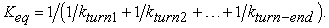(21)

4.2.1. Calculating stiffness of some sample protein molecules

Now we can use the approach discussed in previous section to calculate the stiffness of several protein molecules. The proteins in this numerical experiment were chosen such that empirical or computed data on their stiffness is readily available in the literature. Secondly, we chose proteins with helical structures because of their specific geometry and arrangement of hydrogen bonds. The helical motif can greatly simplify the evaluation of protein stiffness since hydrogen bonds are placed almost parallel to the helix axes. Table 4 lists three sample proteins with the required specification, their PDB (Protein Data Bank) code, the number of amino acids and the number of detected hydrogen bonds (based on the geometric and energetic criteria reported in ). The first molecule is a synthetic peptide, cysteine - lysine - cysteine (C3K30C) specifically designed to study hydrogen bonding by . Under the experimental conditions  this synthetic peptide adopts the a helix structure as a result of hydrogen bonding (31 bonds) within the molecule. The peptide has a length of 53Å and a diameter of approximately 15Å. The stiffness of this protein molecule was measured using atomic force microscopy. Each molecule was stretched from the a-helical state into a linear chain. The stiffness was found to vary with molecule displacement. In order to conduct a meaningful comparison between our calculated stiffness of this protein molecule and the empirical measurement reported in , only small displacements are considered. In particular, we concentrate on deformation of 0.12Å or smaller for the subject protein. With such deformation protein molecule will keep its helical shape and none of the hydrogen bonds breaks. The reported stiffness of this protein molecule varies between 0.3-0.4N/m. Results of our computational analysis applied on this protein molecule are summarized as follows. 31 hydrogen bonds are detected within this protein molecule.

The stiffness of the studied molecule (0.38N/m) is in agreement with the expected range of the stiffness of this molecule ((0.3-0.4N/m)) from empirical data.

The elastic properties of characterized myosin II S2 sub domain using molecular dynamics and normal mode analysis has been studied . Tis protein molecule with 1NKN PDB code, is 87 residue long a-helix. We predicted 146 hydrogen bonds within this molecule structure and 0.083N/m stiffness. This results are in good agreement with the stiffness predicted by  for this protein molecule (0.06-0.08N/m).

To study elasticity and strength of secondary structures of protein molecules  compared the force-extension behavior of α-helices, β-sheets and tropocollagen domains in protein molecules. Their results are provided by using atomistic modeling of nano-mechanical response of the protein molecules at ultra-slow deformation rates. The stiffness predicted for a molecule with helical structure (1GK6) in this study is 0.571N/m. The predicted stiffness for this molecule using our proposed method is 0.52N/m.

### 5. Discussion and Conclusion

This paper presents a novel methodology to study mechanical properties of protein molecules in atomic level. Two major results of this research are: (1) estimating the stiffness of the hydrogen bonds at any given direction and; (2) predicting the stiffness of protein molecules (helical structure). We achieve this through developing equivalent system of mechanical elements (three linear springs) that mimic physical behavior of the actual bonding arrangements. The empirical value of energy is obtained through the energy function of hydrogen bonds reported in literature. Then the stiffness of each spring is calculated based on this empirical data. To obtain these results we solve an optimization problem for each individual hydrogen bond to make sure that both mechanical model and the actual bond have similar potential energies, and change of potential energies through small displacements. Once the mechanical properties of each spring are obtained, the stiffness ellipsoid of the bond is calculated. Having the stiffness ellipsoid one can calculate the stiffness on hydrogen bonds at any given direction. The comparison between stiffness of some sample hydrogen bonds (average of 3.43N/m and standard deviation of 1.3N/m) versus the expected value from literature (3-6N/m) showed that our results are in the expected range.

Furthermore, to obtain the stiffness of helical protein molecules a mechanical model based on hydrogen bonds particular geometry and arrangement is proposed. This methodology is employed on three different helical protein molecules with reported stiffness in literature. The obtained stiffness for these protein molecules are in good agreement with empirical and computational values reported in literature.

In addition further analysis on bond’s directional stiffness combined with the proposed mechanical model of α helices, suggest that the α helices are stiffer in axial direction than in the lateral one. This provides direct mathematical proof to this behavior of helical protein molecules.

This mechanical model to calculate protein molecules stiffness is developed for the helical molecules. Developing a more elaborate mechanical model of the protein molecules will result in the prediction of the stiffness of protein molecules with other structure (e.i. β-sheets).

To the best of our knowledge the analysis reported here is the first direct mechanical analysis of the directional stiffness of individual hydrogen bonds. This analysis, combined with a mechanical model of the arrangement of hydrogen bonds in protein molecules, provides us with a multiscale model to calculate mechanical properties of biological materials starting from atomic levels.

### Acknowledgements

The support provided by National Science Foundation, University of Connecticut and Manhattan College are greatly acknowledged.

### References

  Buehler, M. J., and Keten, S., 2008. “Elasticity, strength and resilience: a comparative study on mechanical signatures of a-helix, -sheet and tropocollagen domains”. Nano Research, 1, pp. 63-71.In article CrossRef  Kazerounian, K., 2004. “From mechanisms and robotics to protein conformation and drug design”. Journal of Mechanical Design, 126, pp. 40-45.In article CrossRef  Hu, X., Cebe, P., Weiss, A. S., Omenetto, F., and Kaplan, D. L., 2012. “Protein-based composite materials”. Materials Today, 15(5), pp. 208-215.In article CrossRef  Rief, M., Gautel, M., Oesterhelt, F., Fernandez, J. M., and Gaub, H. E., 1997. “Reversible unfolding of individual titin immunoglobulin domains by afm”. Science, 276, p. 1109.In article CrossRef PubMed  Gabovich, A. M., and Li, M. S., 2009. “Mechanical stability of proteins”. Journal of Chemical Physics, 131, p. 024121.In article CrossRef PubMed  Lantz, M. A., Jarvis, S. P., Tokumoto, H., Martynski, T., Kusumi, T., Chikashi, N., and Miyake, J., 1999. “Stretching the a-helix: A direct measure of the hydrogen bond energy of a single peptide molecule”. Chemical Physics Letters, 315, pp. 61-68.In article CrossRef  Tskhovrebova, L., Trinick, K., Sleep, J., and Simmons, M., 1997. “Elasticity and unfolding of single molecules of the giant muscle protein titin”. Nature, 387, p. 308.In article CrossRef PubMed  Kellermayera, M. S. Z., Smithb, S. B., Bustamanteb, C., and Granzierc, H. L., 1998. “Complete unfolding of the titin molecule under external force”. Journal of Structural Biology, 122, pp. 197-205.In article CrossRef PubMed  Adamovic, I., Mijailovich, S. M., and Karplus, M., 2008. “The elastic properties of the structurally characterized myosin ii s2 subdomain: A molecular dynamics and normal mode analysis”. Biophysical Journal, 94, pp. 3779-3789.In article CrossRef PubMed  Buehler, M., and Keten, S., 2008. “Elasticity, strength and resilience: A comparative study on mechanical signatures of a helix, b-sheet and tropocollagen domains”. Nano Res, 1, pp. 63-71.In article CrossRef  Hamdia, M., Ferreiraa, A., Sharmab, G., and Mavroidis, C., 2008. “Prototyping bio-nanorobots using molecular dynamics simulation and virtual reality”. Microelectronics Journal, 39, p. 190201.In article CrossRef  Poursina, M., Bhalerao, K. D., Anderson, K. S., Flores, S., and Laederach, A. “Strategies for articulated multibodybased adaptive coarse grain simulation of rna”. Methods in Enzymology, 487(31), pp. 73-98.In article PubMed  Shahbazi, Z., 2011. “Role of hydrogen bonds in kinematic mobility and elasticity analysis of protein molecules”. PhD thesis, University of Connecticut.In article  Kazerounian, K., and H., I., 2012. 21st Century Kinematics. Springer, ch. Protein Molecules: Evolution’s Design for Kinematic Machines, pp. 217-244.In article  Kazerounian, K., Latif, K., and Alvarado, C., 2005. “Protofold: A successive kinetostatic compliance method for protein conformation prediction”. Journal of Mechanical Design, 127(4), pp. 712-717.In article CrossRef  Kazerounian, K., Latif, K., Rodriguez, K., and Alvarado, C., 2005. “Nano-kinematics for analysis of protein molecules”. Journal of Mechanical Design, 127(4), pp. 699-711.In article CrossRef  Subramanian, R., and Kazerounian, K., 2007. “Kinematic mobility analysis of peptide based nano-linkages”. Mechanism and Machine Theory, 42(8), pp. 903-918.In article CrossRef  Subramanian, R., and Kazerounian, K., 2007. “Improved molecular model of a peptide unit for proteins”. Journal of Mechanical Design, 129(11), pp. 1130-1136.In article CrossRef  Shahbazi, Z., Ilies, H., and Kazerounian, K., 2010. “Hydrogen bonds and kinematic mobility of protein molecules”. Journal of Mechanisms and Robotics, 2, pp. 021009-1,9.In article  Shahbazi, Z., and Demirtas, A., 2015. “Rigidity analysis of protein molecules”. Journal of Computing and Information Science in Engineering.In article CrossRef  Z., S., F., P. T. A. P., H., I., K., K., and P., B., 2010. Advances in Robot Kinematics, Issue on Motion in Man and Machine. Springer, ch. A Kinematic Observation and Conjecture for Stable Construct of a Peptide Nanoparticle, pp. 203-210.In article  Wales, T. E., and Fitzgerald, M. C., 2001. “The energetic contribution of backbone–backbone hydrogen bonds to the thermodynamic stability of a hyperstable p22 arc repressor mutant”. J Am Chem Soc, 123(31), pp. 7709-10.In article CrossRef PubMed  Dahiyat, B. I., Gordon, B., and Mayo, S. L., 1997. “automated design of the surface positions of protein helices”. Protein Science, 6, pp. 1333-1337.In article CrossRef PubMed  Jacobs, D., Rader, A. J., Kuhn, L. A., and Thorpe, M. F., 2001. “Protein flexibility predictions using graph theory”. Proteins: Structure, Function, and Genetics, 44(2), pp. 150-165.In article CrossRef PubMed  Baker, E. N., and Hubbard, R. E., 1984. “Hydrogen bonding in globular proteins”. Prog Biophys Mol Biol, 44(2), pp. 97-179.In article CrossRef  Artymiuk, P. J., and Blake, C. C., 1981. “Refinement of human lysozyme at 1.5 a resolution analysis of non-bonded and hydrogen-bond interactions”. J Mol Biol, 152(4), pp. 737-62.In article CrossRef  Xu, D., Tsai, C. J., and Nussinov, R., 1997. “Hydrogen bonds and salt bridges across protein-protein interfaces”. Protein Engineering, 10(9), pp. 999-1012.In article CrossRef PubMed  Brown, I. D., 2006. The chemical bond in inorganic chemistry: the bond valence model. Oxforf University Press.In article CrossRef  Mitchel, B. S., 2003. An introduction to materials engineering and science. John Wiley & Sons, INC.In article  Arora, J. S., 2004. Introduction to optimum design. Elesevier Academic Press.In article  Ashby, M., Shercliff, H., and Cebon, D., 2007. Materials: engineering, science, processing and design. Butterworth-Heinemann.In article  Branden, C., and Tooze, J., 1999. Introduction to protein structure, second ed. Garland publishing.In article  Ackbarow, T., Chen, X., Keten, S., and Buehler, M. J., 2007. “Hierarchies, multiple energy barriers, and robustness govern the fracture mechanics of alpha-helical and beta-sheet protein domains”. Proc Natl Acad Sci U S A, 104(42), pp. 16410-5.In article CrossRef PubMed